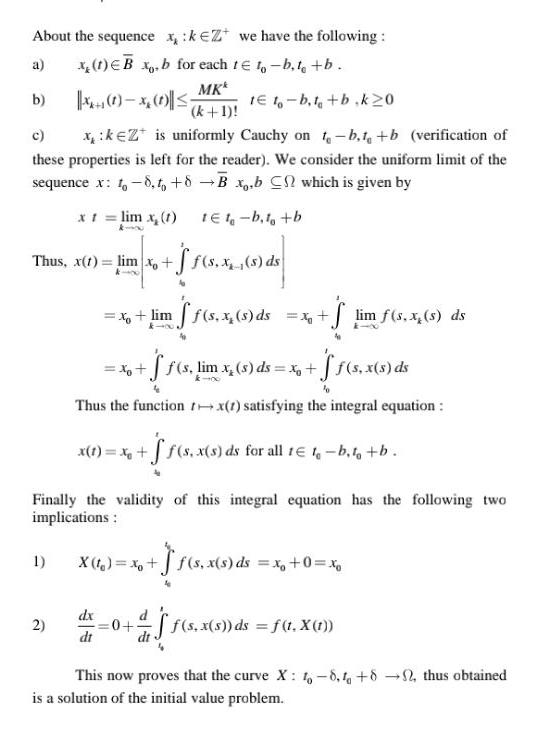Question:

# About the sequence x kezt we have the following a x 1 B x b

Last updated: 9/18/2023About the sequence x kezt we have the following a x 1 B x b for each t 10 b t b MK x x k 1 b c x kezt is uniformly Cauchy on t b t b verification of these properties is left for the reader We consider the uniform limit of the sequence x to 8 to 8 B x b C which is given by x t lim x 1 1E1 b t b Thus x 1 lim xo f s x s ds 1 1 1 b t b k20 Finally the validity of this integral equation has the following two implications X f x f s x s ds x 0 x 2 x lim ff s x s ds x lim f s x s ds x f f s lim x s ds x ff s x s ds Thus the function tx 1 satisfying the integral equation x 1 x f f s x s ds for all 1 t b t b dx dt 0 aff s x s ds f t X 1 This now proves that the curve X 1 8 4 8 thus obtained is a solution of the initial value problem